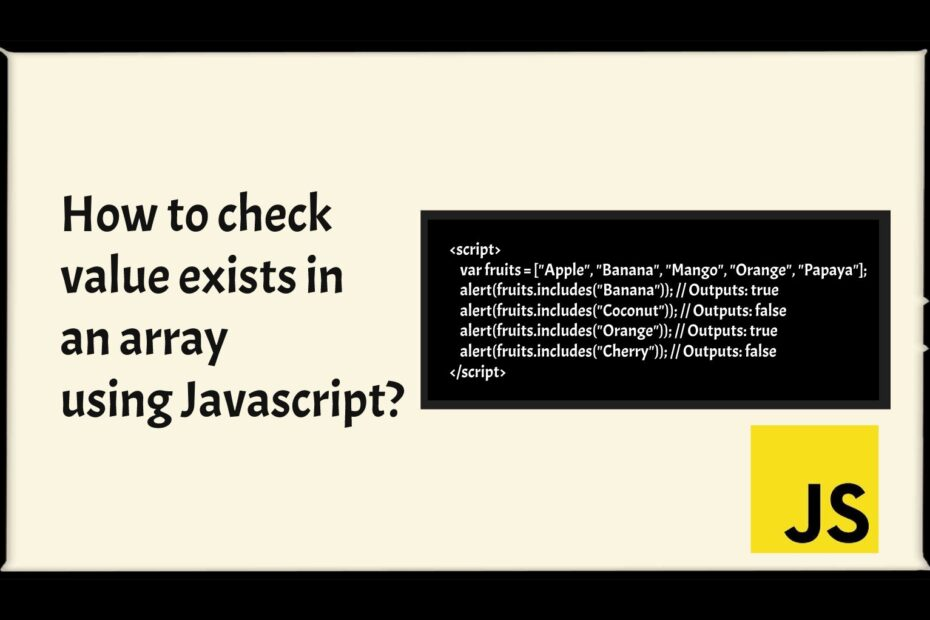# How to check value exists in an array using Javascript?In this tutorial you will going to lean some method to check value exists in an array using JavaScript.

## Method 1 : Use the indexOf()

You can use the indexOf() method to check whether a specific value exists in an array or not. The indexOf() method returns the index of the element within the array if found, and -1 if not found.

Let’s look at the following example for better understand:

``````<script>
var fruits = ["Apple", "Banana", "Mango", "Orange", "Papaya"];

// Check if a value exists in the fruits array
if(fruits.indexOf("Mango") !== -1){
} else{
}
</script>``````

## Method 2 : Use the include()

ES6 introduced include() method to value exists in an array very easy. However, this method only returns true or false instead of the index number, as you can see here:

``````<script>
var fruits = ["Apple", "Banana", "Mango", "Orange", "Papaya"];
</script>``````

## Method 3 : Old JavaScript Loop 🙁

You can also use the for loop to check value exists in an array. This method little old but you can also do this.

``````<script type = 'text/javascript' >

//code to check if a value exists in an array using javascript for loop
var fruits_arr = ['Apple', 'Mango', 'Grapes', 'Orange', 'Fig', 'Cherry'];

function checkValue(value, arr) {
var status = 'Not exist';

for (var i = 0; i < arr.length; i++) {
var name = arr[i];
if (name == value) {
status = 'Exist';
break;
}
}

return status;
}

console.log('status : ' + checkValue('Mango', fruits_arr));
console.log('status : ' + checkValue('Peach', fruits_arr)); ``````

## Check if an array contains an object

Suppose sometime you want to find array contains an object that time you find difficulty how you can do this. This time you can use following method using include()

``````const john = {
'name': 'John Doe',
'email': '[email protected]'
};
const jane = {
'name': 'Jane Doe',
'email': '[email protected]'
};

const list = [john, jane];
let result = list.includes(john);

console.log(result); // true``````

In the above example, the list.includes(john) has returns true because the list array has or contains the john object reference.

Suppose you want o find array within a object using value not with the full object that time you have to write one more function for check equal first and second value. Following is the full example

``````const list = [{
'name': 'John Doe',
'email': '[email protected]'
}, {
'name': 'Jane Doe',
'email': '[email protected]'
}];

const isEqual = (first, second) => {
return JSON.stringify(first) === JSON.stringify(second);
}

const result = list.some(e => isEqual(e, {
'name': 'John Doe',
'email': '[email protected]'
}));

console.log(result); // true``````

Interview Questions for Laravel Developer

Reverse Number in PHP

What is useQuery in react